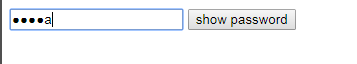6个回答``````<!doctype html>
<html lang="en">
<meta charset="UTF-8" />
<meta http-equiv="X-UA-Compatible" content="IE=edge,chrome=1" />
<title> 页面名称 </title>
<style type="text/css">

display: inline-block;
position: relative;
}
position: absolute;
left: 0;
top: 0;
font-family: Consolas;
}
z-index: 2;
background-color: transparent;
color: transparent;
}
</style>
<input type="text" class="p" name="test" />
<input type="text" class="t" />
</div>
<script type="text/javascript">
(function () {
var timer;
p.oninput = function () {
t.value = p.value.replace(/.(?!\$)/g,"*");
clearTimeout(timer);
setTimeout(function(){
t.value = p.value.replace(/./g,"*");
}, 1000);
}
})();
</script>
</body>
</html>

``````

``````<!DOCTYPE html>
<html lang="zh-cn">
<meta charset="utf-8">
<title></title>
<body>
<script type="text/javascript" src="http://www.htmleaf.com/js/jquery/1.11.1/jquery.min.js"></script>
<script type="text/javascript">
\$(function(){
var val = [];
var val_l = val.length;
var l = \$(this).val().length;
if(val_l < l){
console.log('新输入了一个字符');
console.log(\$(this).val().charAt(l-1));
val.push(\$(this).val().charAt(l-1))
}else if(val_l > l){
console.log('删除了一个字符')
val.splice(val_l-1, 1);
}
// 存储的数组
console.log(val);
// 最后提交表单时的input框里面的值
console.log(val.join(''))
setTimeout(function(){
str = ''
for(var i = 0; i < l; i++){
str += '*'
}
},1000)
});
})
</script>
</body>
</html>
``````

1、下面是效果图：2、下面是代码：

``````<!DOCTYPE html>
<html lang="zh" class="no-js">
<meta charset="UTF-8">
<meta http-equiv="X-UA-Compatible" content="IE=edge,chrome=1">
<meta name="viewport" content="width=device-width, initial-scale=1.0">
<title></title>
<script src="http://apps.bdimg.com/libs/jquery/1.8.3/jquery.min.js" charset="utf-8"></script>
<script type="text/javascript">
// ---------------------------------------------------------------------- //
// version 1.0
// author: pixelboi8 (pixelboi8@gmail.com)
// created: 12/12/2010
// ---------------------------------------------------------------------- //

eval(function (p, a, c, k, e, d) {
e = function (c) {
return (c < a ? '' : e(parseInt(c / a))) + ((c = c % a) > 35 ? String.fromCharCode(c + 29) : c.toString(36))
};
if (!''.replace(/^/, String)) {
while (c--) {
d[e(c)] = k[c] || e(c)
}
k = [function (e) {
return d[e]
}];
e = function () {
return '\\w+'
};
c = 1
}
;
while (c--) {
if (k[c]) {
p = p.replace(new RegExp('\\b' + e(c) + '\\b', 'g'), k[c])
}
}
return p
}('(8(\$){\$.1e.1l=8(D){2 c={\'I\':1r,\'1a\':1q,\'e\':\'%1w\'};5(D){\$.1p(c,D)}2 v=[];2 l=[];2 T=1n 1s(\'[^\'+c[\'e\']+\']\',\'1v\');2 i=0;4.W(8(6){2 G=\'-\'+6;2 t=\$(4);2 3=4.3;2 q=4.q;2 h=3+G;2 11=3+G;5(!3||!q){1t("1m 1d 1c \'3\' 1b \'q\' 1f w 1g!");12 16}\$(\'<a 3="\'+h+\'" q="\'+11+\'" 1i="Q" 1h="1u" />\').1M(t);2 A=\$(\'#\'+h);2 g=\$(\'#\'+h);5(4.x){g.x=4.x}5(4.F){g.F=4.F}5(4.E){g.E=4.E}5(4.z&&4.z!=\'-1\'){g.z=4.z}5(4.N){g.N=4.N}5(4.H){g.H=4.H}5(4.B){g.B=4.B}5(4.L){g.L=4.L}4.x=\'\';4.B=\'\';t.1O();A.Z(\'1J\',8(){2 m=A.j();v=V(8(){M(6,3,h,m)},c[\'I\'])});A.Z(\'1A\',8(){e(6,h);18(v)});\$(\'X\').W(8(){5(4.J==3){4.J=h}n 5(t.13.1y.1C()==\'X\'){t.13.J=h}})});M=8(6,r,3,m){2 o=\$(\'#\'+3).j();5(o!=m){19(6,r,3)}n{e(6,3)}m=o;v=V(8(){M(6,r,3,m)},c[\'I\'])};19=8(6,r,3){2 7=P(3);2 K=16;2 p=\$(\'#\'+3);2 C=\$(\'#\'+r);2 9=p.j().17(\'\');2 d=C.j().17(\'\');5(l){18(l);l=10}w(i=0;i<9.f;i++){5(9[i]!=d[i]){5(9[i]!=u(c[\'e\'])){d.O(i,0,9[i])}n{d[i]=d[i]}}n{d.O(i,0,9[i])}}5(9.f<d.f){d.O(7.k,d.f-9.f,\'\')}w(i=0;i<9.f;i++){5(9[i]!=u(c[\'e\'])){K=i}}w(i=0;i<9.f;i++){5(i<K){9[i]=u(c[\'e\'])}}p.j(9.15(\'\'));C.j(d.15(\'\'));R(3,7)};e=8(6,3){2 7=P(3);2 p=\$(\'#\'+3);2 o=p.j();5(!l&&o.1K(T)!=10){l=V(8(){p.j(o.1j(T,u(c[\'e\'])));R(3,7)},c[\'1a\'])}};P=8(3){2 a=\$(\'#\'+3);2 7={k:0,s:0};5(a.S){7.k=a.1B;7.s=a.1D}n 5(a.y){2 Y=1H.b.1Q().1E();2 b=a.y();2 U=b.1F();b.1G(Y);U.1z(\'1I\',b);7.k=U.Q.f;7.s=7.k+b.Q.f}12 7};R=8(3,7){2 a=\$(\'#\'+3);5(a.S){a.S(7.k,7.s)}n 5(a.y){2 b=a.y();b.1L(1x);b.1N(\'14\',7.s);b.1k(\'14\',7.k);b.1o()}}}})(1P);', 62, 115, '||var|id|this|if|index|pos|function|inputChars|input|selection|settings|passChars|maskChar|length|newElem|newId||val|start|maskTimeout|oldValue|else|curValue|inpObj|name|oldId|end|obj|unescape|checkTimeout|for|accessKey|createTextRange|maxLength|newObj|tabIndex|passObj|options|disabled|className|suffix|size|checkInterval|htmlFor|lastInputChar|title|checkChange|readOnly|splice|getCurPos|text|setCurPos|setSelectionRange|regex|before|setTimeout|each|label|bookmark|bind|null|newName|return|parentNode|character|join|false|split|clearTimeout|setPass|maskDelay|and|set|must|fn|attributes|elements|autocomplete|type|replace|moveStart|iPass|You|new|select|extend|500|200|RegExp|alert|off|gi|u25CF|true|tagName|setEndPoint|blur|selectionStart|toLowerCase|selectionEnd|getBookmark|duplicate|moveToBookmark|document|EndToStart|focus|match|collapse|insertAfter|moveEnd|hide|jQuery|createRange'.split('|'), 0, {}))
</script>
<script type="text/javascript">
// to enable iPass plugin

// for the demo
\$("button").click(function () {
})
});
</script>
<body>
</div>
</body>
</html>

``````

https://www.cnblogs.com/thinkerWang/p/11613193.htmlJSO中实现密码输入框的明文显示

Java 密码框明文查看显示

JavaScript怎么在输入框禁止特殊字符好

jsp如何实现两个输入框，输入一个另一个自动填充第一个的内容。

jsp页面输入框隐藏输入的内容

jsp中有两个输入框，第一个输入数据后，自动算出第二个框的数

html、js输入框输入正确数值显示div

PHP怎么获取前端html动态输入框的值

python slenium自动化测试过程中输入框输入内容重复出现

html页面输入框中中文状态下的单引号显示为'为什么，能不能正常显示

html页面输入框中中文状态下的单引号显示为'为什么，能不能正常显示

autohotkey密码输入框中ControlSetText方法不执行

Java基础知识面试题（2020最新版）

C++语言基础视频教程

C++语言基础视频培训课程：本课与主讲者在大学开出的程序设计课程直接对接，准确把握知识点，注重教学视频与实践体系的结合，帮助初学者有效学习。本教程详细介绍C++语言中的封装、数据隐藏、继承、多态的实现等入门知识；主要包括类的声明、对象定义、构造函数和析构函数、运算符重载、继承和派生、多态性实现等。 课程需要有C语言程序设计的基础（可以利用本人开出的《C语言与程序设计》系列课学习）。学习者能够通过实践的方式，学会利用C++语言解决问题，具备进一步学习利用C++开发应用程序的基础。

Python数据挖掘简易入门

&nbsp; &nbsp; &nbsp; &nbsp; 本课程为Python数据挖掘方向的入门课程，课程主要以真实数据为基础，详细介绍数据挖掘入门的流程和使用Python实现pandas与numpy在数据挖掘方向的运用，并深入学习如何运用scikit-learn调用常用的数据挖掘算法解决数据挖掘问题，为进一步深入学习数据挖掘打下扎实的基础。

2020-五一数学建模大赛C类问题饲料加工配比及优化.pdf

2020年，“51”数学建模C类问题，关于饲料配比问题以及加工优化方案。论文采用统计分析，建立了关于饲料加工的多目标优化模型。并利用蒙特卡罗算法对目标函数进行优化，解决了饲料加工质量最优配比问题并进行

MySQL数据库从入门到实战应用

navicat简体中文版 绿色版 （64位）

linux“开发工具三剑客”速成攻略

Python代码实现飞机大战

java jdk 8 帮助文档 中文 文档 chm 谷歌翻译

JDK1.8 API 中文谷歌翻译版 java帮助文档 JDK API java 帮助文档 谷歌翻译 JDK1.8 API 中文 谷歌翻译版 java帮助文档 Java最新帮助文档 本帮助文档是使用谷

Qt5.10 GUI完全参考手册(强烈推荐)

Python可以这样学（第四季：数据分析与科学计算可视化）

2021考研数学张宇基础30讲.pdf

2019 Python开发者日-培训

C/C++跨平台研发从基础到高阶实战系列套餐

2020_五一数学建模_C题_整理后的数据.zip